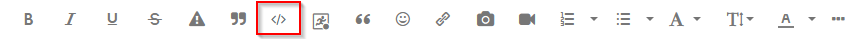## proc gplot

Hi!

I'm trying to create a plot where BMI is the vaxis and Age_grp is the horinzontal axis.

I'm getting an error message in the log: Error: At least one PLOT or BUBBLE statement must be given.

This is my program below:

proc gplot data=mclib.bmi;
plot bmi*age_gp
vaxis=axis2 haxis= axis1;
symbol1 v=dot cv=red w=0.1;
axis1 order=('Middle' 'Old' 'Young')
label=(font="arial" "Age group");
axis2 order=(15,20,25,30,35,40)
label=(font="arial" angle=90 "Body Mass Index");
run;
quit;

2 REPLIES 2

## Re: proc gplot

Please examine your code very very carefully. You are missing a semi-colon in a key place.

By the way, @PrinceAde , from now on, please show us the ENTIRE log for a given PROC if there is an error message. Do not show us just the error messages. Please copy the log as text and click on the </> icon and paste the log into the window that appears.--
Paige Miller

## Re: proc gplot

Hi!

I'm trying to create a plot where BMI is the vaxis and Age_grp is the horinzontal axis.

I'm getting an error message in the log: Error: At least one PLOT or BUBBLE statement must be given.

This is my program below:

proc gplot data=mclib.bmi;
plot bmi*age_gp
vaxis=axis2 haxis= axis1;
symbol1 v=dot cv=red w=0.1;
axis1 order=('Middle' 'Old' 'Young')
label=(font="arial" "Age group");
axis2 order=(15,20,25,30,35,40)
label=(font="arial" angle=90 "Body Mass Index");
run;
quit;

My guess. Look at the highlighted text above.

But I think this is not the first time it has been recommended to provide the LOG and all the notes, messages and warnings.

Discussion stats
• 2 replies
• 111 views
• 0 likes
• 3 in conversation